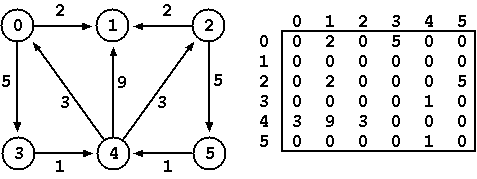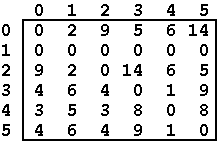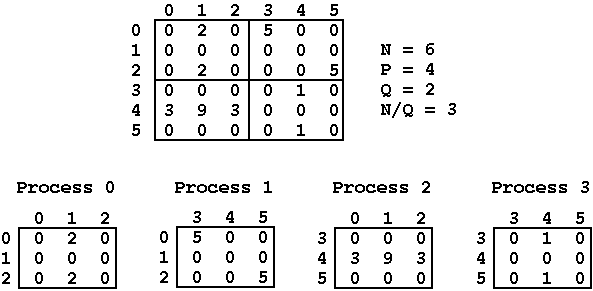# Assignment I: All-Pairs Shortest Path Problem

## Goal

Allow students to acquire sensibility and practical experience with parallel programming for distributed memory environments using the MPI framework.

## Description

The problem to be solved consists in determining all shortest paths between pairs of nodes in a given graph. This is the well known all-pairs shortest path problem.

The idea is simple: given a directed graph G = (V,E) in which V is the set of nodes (or vertices) and E is the set of links (or edges) between nodes, the goal is to determine for each pair of nodes (vi,vj) the size of the shortest path that begins in vi and ends in vj. A path is simply a sequence of links in which the end node and start node of two consecutive links are the same. One link can be seen as a tuple of the form <vi, vj, t> where vi is the origin node, vj is the destination node and t is the size of the link between the nodes. The sum of the sizes of the links is the size of the path between vi and vj.

## Implementation

A directed graph with V nodes can be represented as a matrix D1 with dimension NxN (N = 6 for the example that follows) where each element (i,j) of the matrix represents the size of the link that connects node vi with node vj. A zero value means that there is no connection between the two nodes.The solution of the problem may also be represented by a matrix Df with dimension NxN where each element (i,j) of the matrix corresponds to the size of the shortest path among all possible paths between nodes vi and vj.Min-plus matrix multiplication, also known as Distance Product, is an adapted form of matrix multiplication in which the multiplication and sum operations are replaced by operations of sum and minimum, respectively. Using this min-plus matrix multiplication strategy, the Repeated Squaring algorithm allows one to obtain the matrix Df from matrix D1 by building successively matrices D2 = D1xD1, D4 = D2xD2, D8 = D4xD4, ..., until Df = DgxDg, with f >= N and g < N (see here a more detailed explanation of this algorithm).

A well-known algorithm to efficiently compute the multiplication of matrices with the same size in distributed environments is Fox's algorithm. The application of Fox's algorithm is not always possible, it depends on the dimension of the matrices being multiplied and on the available processes. Given matrices of size NxN and P processors, there must exist an integer Q such that P = Q*Q and N mod Q = 0, otherwise the algorithm cannot be applied. Fox's algorithm then divides the NxN matrices into sub-matrices with dimension (N/Q)x(N/Q) and assigns each one to the P processors available in the system.To compute the result of each sub-matrix multiplication, each process only needs to exchange information with the processes in the same row and same column of its sub-matrix, thus reducing the number of messages that need to be exchanged (see pages 29 and 30 of the A Users' Guide to MPI for a more detailed explanation of Fox's algorithm). Applying successively the Fox algorithm allows one to calculate matrices D2, D4, ..., Df of the Repeated Squaring algorithm and thus obtain the matrix that is the solution to our problem.

## Input and Output

The application to be developed must accept as input a file with the description of the graph to be considered. Such description must obey the following pattern: the first input line has the integer N representing the number of nodes in the graph, followed by N lines each representing the links between pairs of nodes of the graph (similarly to the matrices shown above). For instance, the input file describing the graph in our example looks as follows:
```6
0 2 0 5 0 0
0 0 0 0 0 0
0 2 0 0 0 5
0 0 0 0 1 0
3 9 3 0 0 0
0 0 0 0 1 0
```
If the dimension of the matrix and the number of available processes are not compatible with the conditions of the Fox algorithm, you must provide an appropriate error message and the execution must be canceled.

As output, your application must print the Df matrix representing the solution for the problem, one row per line. For the given example, the output should be:

```0 2 9 5 6 14
0 0 0 0 0 0
9 2 0 14 6 5
4 6 4 0 1 9
3 5 3 8 0 8
4 6 4 9 1 0
```
The input file must be read from the standard input and the results are to be printed to the standard output (click here to get a set of test examples).

## What to Deliver?

A ZIP file including:
• All source code files (can be just one) implementing your solution
• A makefile that generates the executable program (assign name fox)
• Short technical report (no more than 5 pages) in PDF format describing the work done (free structure) and including:
• A summary on the algorithm implementation (include details about the base idea of the algorithm, main data structures, relevant auxiliary functions, type of communications, ...)
• Performance evaluation including execution times (in milliseconds and excluding I/O) and speedups (comparing with sequential execution and with execution with 1 process) for, at least, 1, 4, 9 and 16 processes
• Main difficulties encountered in the development of the work (if any) and comments/suggestions about the work (optional)
The code must compile and execute in the machines made available in Lab1 and that constitute your cluster. Legibility and code structure will be valued for the final mark.

The ZIP file (containing the items described above) must be sent to miguel-areias@dcc.fc.up.pt until 8th of November and presented orally in the week after (15th of November, time to be specified).

## Cluster Setup

To avoid having to authenticate at each node every time you run MPI, do the following:
• Generate an authentication key with the command

ssh-keygen -t rsa

This will produce two files in your home directory:

~/.ssh/id_rsa with your private key, and